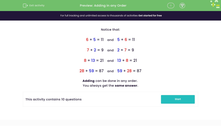# Understand that Adding Can be Done in any Order

In this worksheet, students will show understanding that addition can be done in any order.Key stage:  KS 1

Curriculum topic:   Number: Addition and Subtraction

Curriculum subtopic:   Understand Order for Addition/Subtraction

Difficulty level:#### Worksheet Overview

Take a look at these addition facts below:

6 + 5 = 11    and    5 + 6 = 11

7 + 2 = 9    and    2 + 7 = 9

8 + 13 = 21    and    13 + 8 = 21

28 + 59 = 87    and    59 + 28 = 87

Did you spot that adding can be done in any order?

The answer will be the same!Let's test it out in these questions.

### What is EdPlace?

We're your National Curriculum aligned online education content provider helping each child succeed in English, maths and science from year 1 to GCSE. With an EdPlace account you’ll be able to track and measure progress, helping each child achieve their best. We build confidence and attainment by personalising each child’s learning at a level that suits them.

Get started